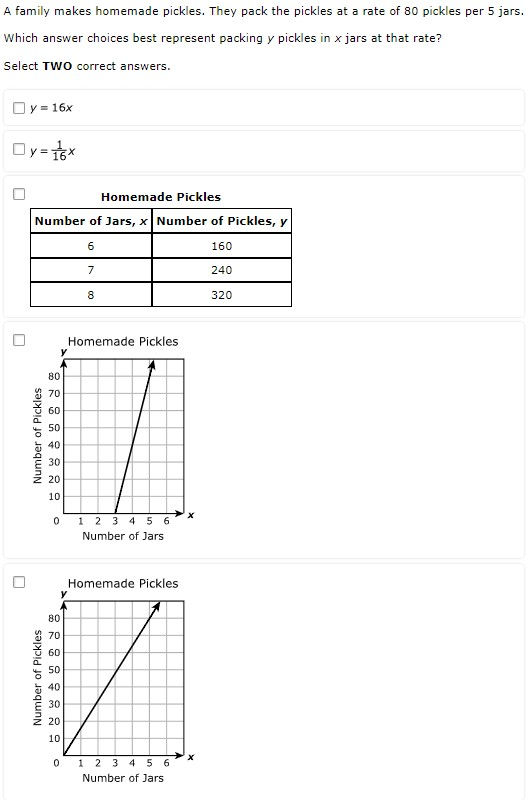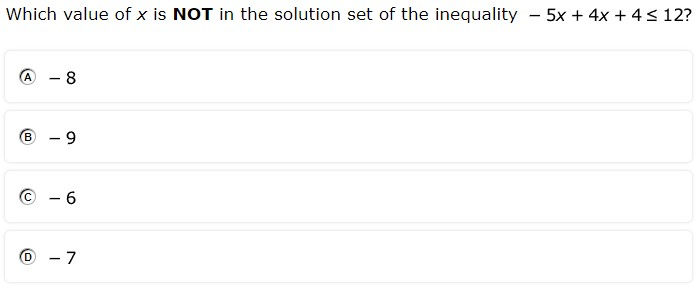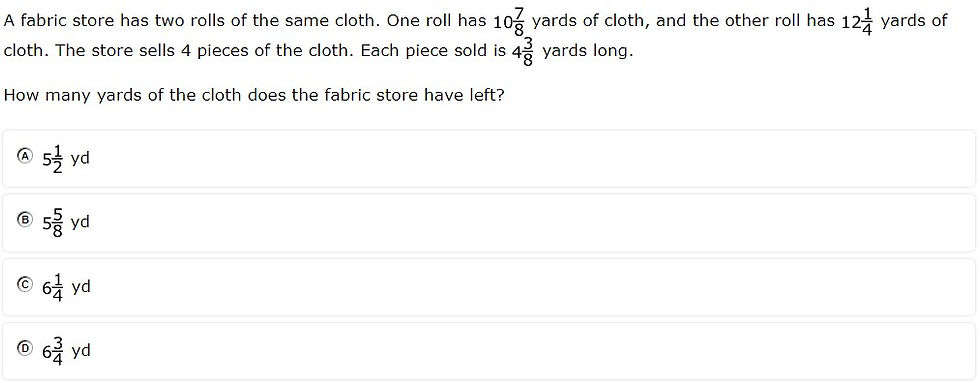top of page
Search

# Lessons learned from the 2023 7th Grade Math STAAR

Updated: Nov 12

The 2023 7th Grade Math STAAR introduced statewide online testing and several new item types. Using a modified version of the statewide item analysis report, I examined the readiness standards that had less than 50% mastery. Each standard has both an analysis of the items themselves to infer what made them so difficult and instructional implications for educators to ensure a more successful 2024 STAAR test.

 Standard # of items % mastery 7.6G 2 28.5 7.7A 2 32 7.9C 2 34 7.6I 2 36 7.4A 2 40.5 7.12A 2 42.5 7.11A 2 42.5 7.4D 2 44 7.9B 2 45.5 7.3B 2 47.5 7.9A 2 49

Access the slide deck here.

### 7.6G - 28.5% overall mastery

solve problems using data represented in bar graphs, dot plots, and circle graphs, including part-to-whole and part-to-part comparisons and equivalents

#12 - 32% correct#28 - 25% full credit, 47% partial credit, 28% no credit###### Analysis
• Multiple successive operations used to find percent of a bar graph

• Circle graph had to be converted from percents to actual numbers

• Multiple select (choose three out of five) might have encouraged guessing

###### Instructional Implications
• Practice finding percent of a whole (e.g., What is 16% of 50)?

• When solving bar graphs, practice finding percent of the graph (e.g., 60% of total sales) by using combinations of categories (e.g., brand C and brand B)

• When solving circle graphs, students should fill in the graph with a percent and an actual number for each category before answering the question

### 7.7A - 32% overall mastery

represent linear relationships using verbal descriptions, tables, graphs, and equations that simplify to the form y = mx + b

#7 - 52% correct#25 - 12% full credit, 29% partial credit, 59% no credit###### Analysis
• Slope-intercept form had to be interpreted from a problem situation

• Slope had to be calculated from pairs of coordinates

• Y-intercept had to be recognized from ordered pair where x=0 and used as b

• Equation for slope not on reference materials

###### Instructional Implications
• Practice converting problem situations into slope-intercept form and using the equation to calculate a table of values

• Ensure students know that you can find y-intercept if given an ordered pair with an x-value of 0

• Practice finding slope with m = (y2 - y1)/(x2 - x1)

Watch the full walkthrough of all 38 items on the 2023 7th Grade STAAR below.

### 7.9C - 34% overall mastery

determine the area of composite figures containing combinations of rectangles, squares, parallelograms, trapezoids, triangles, semicircles, and quarter circles

#13 - 32% correct#26 - 38% correct###### Analysis
• Area of a trapezoid formula more complex than rectangle or triangle

• Equation editor provided no confirmation of solution (e.g., from multiple choice)

• Many calculations required for finding area of dark wood

• Answer distribution on #26 suggests guessing

###### Instructional Implications
• Provide many opportunities for finding area of various composite shapes

• Given figure with shaded/unshaded regions, have students set up equations to find the area of both

• Given figure with shaded/unshaded regions, have students find the area of both

### 7.6I - 36% overall mastery

determine experimental and theoretical probabilities related to simple and compound events using data and sample spaces

#16 - 46% correct#30 - 26% correct###### Analysis
• Students had to find experimental probability but theoretical probability was one of the answer choices (chosen by 24%)

• Compound probability confused many students, with the most chosen answer (37%) being the number of choices available (3), a solution that required no calculations

###### Instructional Implications
• Provide students with experimental data and have students find both the experimental and theoretical probabilities (and discuss the differences)

• Have students solve compound probabilities both with a tree diagram and mathematical calculation

### 7.4A - 40.5% overall mastery

represent constant rates of change in mathematical and real-world problems given pictorial, tabular, verbal, numeric, graphical, and algebraic representations, including d = rt

#9 - 38% correct#23 - 43% full credit, 43% partial credit, 13% no credit###### Analysis
• The graphing online tool is not 100% intuitive

• Not visually clear that students can choose ½ as an x-coordinate

• Multiple select allows for greater confidence while guessing

###### Instructional Implications
• Provide students with coordinate grids and have them plot constant rates, providing multiple points and using various scale factors

• Give students constant rate in a problem situation and have them calculate in direct variation form (y=kx), create a table, and graph the equation

### 7.12A - 42.5% overall mastery

compare two groups of numeric data using comparative dot plots or box plots by comparing their shapes, centers, and spreads

#10 - 43% correct#32 - 42% full credit, 17% partial credit, 42% no credit###### Analysis
• Students had to apply various concepts using domain-specific vocabulary terms (e.g., median, range, interquartile range)

• Evaluating statements and finding the one that supports the graph requires up to 4X the calculations

• Interpreting box plots assumes understanding of quartiles

###### Instructional Implications
• In addition to calculating range, IQR, etc., have students find range of individual quartiles (e.g., What’s the range of the 2nd quartile?)

• Median should be calculated on box plots, dot plots, and with raw data (sorted and unsorted)

### 7.11A - 42.5% overall mastery

model and solve one-variable, two-step equations and inequalities

#18 - 38% correct#38 - 47% correct###### Analysis
• Students had to invert the inequality due to dividing by a negative

• Like terms had to be simplified in addition to using properties of equality

• Equation was modeled using algebra tiles rather than being written

###### Instructional Implications
• Provide problems that require students to combine like terms before isolating the variable

• Encourage students to check work with substitution

• Give students an equation or problem situation and have them translate that into a representation using algebra tiles

• unsorted)

### 7.4D - 44% overall mastery

solve problems involving ratios, rates, and percents, including multi-step problems involving percent increase and percent decrease, and financial literacy problems

#1 - 50% correct#21 - 38% correct###### Analysis
• Students had use a ratio to solve a problem situation

• Calculating percent increase requires a specific ratio that is not provided on the reference materials

• The percent increase problem required a calculation before finding the ratio

###### Instructional Implications
• Have students use a ratio to find both items in a problem situation (e.g., find the number of almonds AND raisins)

• Extra practice with percent increase and decrease problems

• Provide opportunities to talk through reasonableness in percent increase or decrease problems (e.g., \$1,560 is a little more than twice \$750)

• unsorted)

### 7.9B - 45.5% overall mastery

determine the circumference and area of circles

#8 - 34% correct#37 - 57% correct###### Analysis
• Students more often chose the circumference (B) than the area (D) because the diameter was given rather than the radius

• When given the radius, students had to choose the correct formula (C = 2πr rather than C = πd)

• Multiplying a decimal without technology (3.14) offers challenges

###### Instructional Implications
• Give students a circle and have them calculate both area and circumference, noting the relationship with the two

• Have students practice estimating the circumference by tripling the diameter or multiplying the radius by 6

• unsorted)

### 7.3B - 47.5% overall mastery

apply and extend previous understandings of operations to solve problems using addition, subtraction, multiplication, and division of rational numbers

#14 - 44% correct#31 - 51% correct###### Analysis
• The addition, subtraction, and multiplication of mixed numbers involved regrouping an improper fraction

• Hidden number (i.e., the number of hours between 4:00 and 10:00) made the problem more complex

###### Instructional Implications
• Reinforce mixed number concepts with concrete and pictorial representations

• Provide ample opportunities for students to add, subtract, and multiply mixed numbers, regrouping the fraction as needed

• Have students practice operational problems that involve time intervals to simulate the hidden number

### 7.9A - 49% overall mastery

solve problems involving the volume of rectangular prisms, triangular prisms, rectangular pyramids, and triangular pyramids

#17 - 39% correct#34 - 59% correct###### Analysis
• Students were given the side length of the base rather than the area of the base

• Rather than solving for volume, students were given volume and solved for height

###### Instructional Implications
• Practice solving for pyramids with a variety of bases (e.g., square, triangle, rectangle), providing only height and side lengths

• Have students solve for multiple dimensions (e.g., base, height, side length) in addition to solving for volume